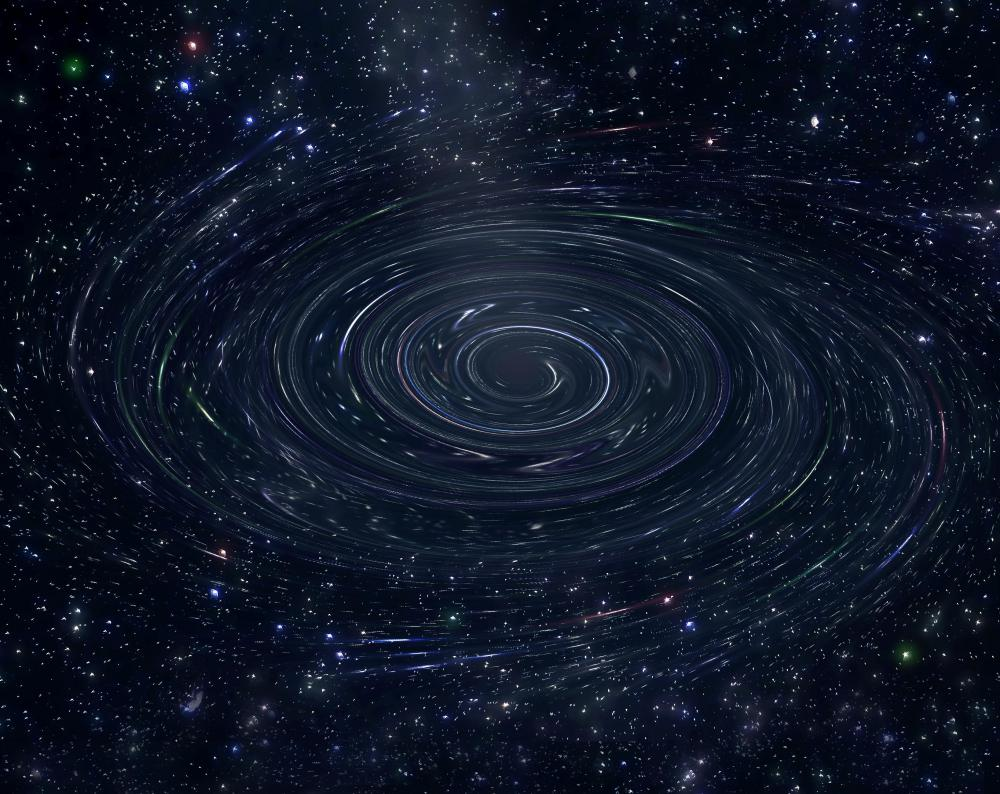# What Is Hydrostatic Equilibrium?

Phil Riddel

A volume of fluid, which can be a gas or a liquid, is said to be in hydrostatic equilibrium when the downward force exerted by gravity is balanced by an upward force exerted by the pressure of the fluid. For example, the Earth’s atmosphere is pulled downwards by gravity, but toward the surface the air is compressed by the weight of all the air above, so the air’s density increases from the top of the atmosphere to the Earth’s surface. This density difference means that air pressure decreases with altitude so that the upward pressure from below is greater than the downward pressure from above and this net upward force balances the downward force of gravity, keeping the atmosphere at a more or less constant height. When a volume of fluid is not in hydrostatic equilibrium it must contract if the gravitational force exceeds the pressure, or expand if the internal pressure is greater.In the case of a black hole, hydrostatic equilibrium cannot be achieved.

This concept can be expressed as the hydrostatic equilibrium equation. It is usually stated as dp/dz = −gρ and applies to a layer of fluid within a larger volume in hydrostatic equilibrium, where dp is the change in pressure within the layer, dz is the thickness of the layer, g is the acceleration due to gravity and ρ is the density of the fluid. The equation can be used to calculate, for example, the pressure within a planetary atmosphere at a given height above the surface.A volume of fluid is in hydrostatic equilibrium when the downward force exerted by gravity is balanced by an upward force exerted by the pressure of the fluid.

A volume of gas in space, such as a large cloud of hydrogen, will initially contract due to gravity, with its pressure increasing toward the center. The contraction will continue until there is an outward force equal to the inward gravitational force. This is normally the point when the pressure at the center is so great that the hydrogen nuclei fuse together to produce helium in a process called nuclear fusion that releases huge amounts of energy, giving birth to a star. The resulting heat increases the pressure of the gas, producing an outward force to balance the inward gravitational force, so that the star will be in hydrostatic equilibrium. In the event of gravity increasing, perhaps through more gas falling into the star, the density and temperature of the gas will also increase, providing more outward pressure and maintaining the equilibrium.

Stars remain in hydrostatic equilibrium over long periods, typically several billion years, but eventually they will run out of hydrogen and begin fusing progressively heavier elements. These changes temporarily put the star out of equilibrium, causing expansion or contraction until a new equilibrium is established. Iron cannot be fused into heavier elements, as this would require more energy than the process would produce, so when all the star’s nuclear fuel has eventually transformed into iron, no further fusion can take place and the star collapses. This might leave a solid iron core, a neutron star or a black hole, depending on the mass of the star. In the case of a black hole, no known physical process can generate sufficient internal pressure to halt gravitational collapse, so hydrostatic equilibrium cannot be achieved and it is thought that the star contracts to a point of infinite density known as a singularity.

## You might also Likeanon999764

This is really informative.